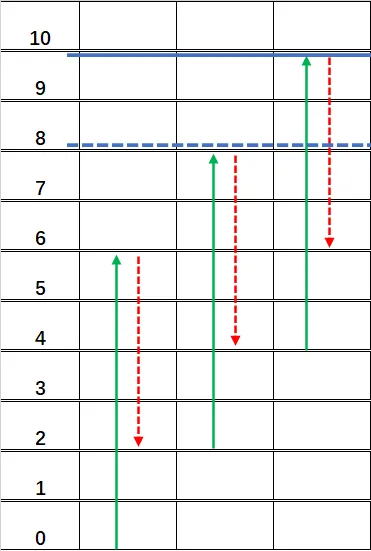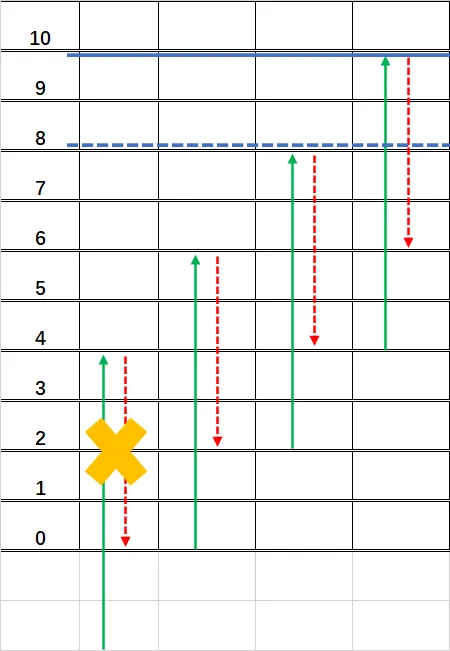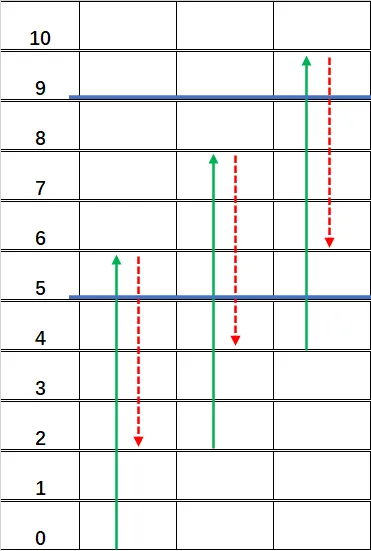CodeTON Round 4 (Div. 1 + Div. 2, Rated, Prizes!)

D. Climbing the Tree

https://codeforces.com/contest/1810/problem/D

2 seconds / 256 megabytes

standard input / standard output

## Problem

The snails are climbing a tree. The tree height is $h$ meters, and snails start at position $0$.

Each snail has two attributes $a$ and $b$ ($a > b$). Starting from the $1$-st day, one snail climbs the tree like this: during the daylight hours of the day, he climbs up $a$ meters; during the night, the snail rests, and he slides down $b$ meters. If on the $n$-th day, the snail reaches position $h$ for the first time (that is, the top of the tree), he will finish climbing, and we say that the snail spends $n$ days climbing the tree. Note that on the last day of climbing, the snail doesn't necessarily climb up $a$ meters, in case his distance to the top is smaller than $a$.

Unfortunately, you don't know the exact tree height $h$ at first, but you know that $h$ is a positive integer. There are $q$ events of two kinds.

• Event of type $1$: a snail with attributes $a$, $b$ comes and claims that he spent $n$ days climbing the tree. If this message contradicts previously adopted information (i. e. there is no tree for which all previously adopted statements and this one are true), ignore it. Otherwise, adopt it.
• Event of type $2$: a snail with attributes $a$, $b$ comes and asks you how many days he will spend if he climbs the tree. You can only give the answer based on the information you have adopted so far. If you cannot determine the answer precisely, report that.

You need to deal with all the events in order.

## Input

Each test contains multiple test cases. The first line contains a single integer $t$ ($1 \le t \le 10^4$) — the number of test cases. Then follows their description.

The first line of each test case contains one integer $q$ ($1\le q \le 2\cdot 10^5$) — the number of events.

For the following $q$ lines, the first integer of each line is either $1$ or $2$, denoting the event type.

If the event type is $1$, then three integers $a$, $b$, and $n$ ($1\le a,b,n \le 10^9$, $a>b$) follow.

If the event type is $2$, then two integers $a$ and $b$ ($1\le a,b \le 10^9$, $a>b$) follow.

It is guaranteed that the sum of $q$ over all test cases does not exceed $2\cdot 10^5$.

## Output

For each test case, output $q$ integers in one line, one for each event, in order. Specifically,

• for each event of type $1$, if you adopt the message, output $1$; if you ignore it, output $0$;
• for each event of type $2$, output an integer denoting the number of days that the snail will spend. If you cannot determine it, output $-1$.

## Example

Input

5
3
1 3 2 5
2 4 1
2 3 2
3
1 6 5 1
2 3 1
2 6 2
3
1 4 2 2
1 2 1 3
2 10 2
9
1 7 3 6
1 2 1 8
2 5 1
1 10 9 7
1 8 1 2
1 10 5 8
1 10 7 7
2 7 4
1 9 4 2
9
1 2 1 6
1 8 5 6
1 4 2 7
2 9 1
1 5 1 4
1 5 2 7
1 7 1 9
1 9 1 4
2 10 8

Output

1 2 5
1 -1 1
1 0 1
1 0 -1 0 0 0 1 8 0
1 0 0 1 0 0 0 0 1

## Note

In the first test case, we can determine $h=7$ through the first message, so we know the second snail and the third snail need to spend $2$ and $5$ days respectively to reach the top.

Let's show how the second snail climbs:

• During the daylight hours of the $1$st day: climbs up $4$ meters, now at position $4$.
• During the night of the $1$st day: slides down $1$ meters, now at position $3$.
• During the daylight hours of the $2$nd day: climbs up $4$ meters, now at position $7$ (reaches the top).

In the third test case, the second snail's message contradicts the first snail's, because the second snail says he spent $3$ days, and he can climb at most $1+1+2=4$ meters in the first $3$ days. However, the first snail only needs $1$ day to climb $4$ meters.

## 题解

#### 整体框架

• 收到信息（事件 $1$）：通过给定的 $a,b,n$，计算出新的树高预测值 $(lb',ub']$，若

• $(lb,ub]\cap(lb',ub']=\varnothing$（即 $ub\leq lb'$ 或 $ub'\leq lb$）：新信息与已有信息矛盾，拒绝该信息。
• $(lb,ub]\cap(lb',ub']\neq\varnothing$：新信息与已有信息不矛盾，接受该信息，更新 $lb=\max(lb,lb'),ub=\min(ub,ub')$.
• 收到询问（事件 $2$）：通过给定的 $a,b$，判断爬到 $lb$ 需要的天数 $d_{min}$，爬到 $ub$ 需要的天数 $d_{max}$，若

• $d_{min}=d_{max}$：则能够确定爬上树需要的天数为 $d_{min}$.
• $d_{min}\neq d_{max}$：无法确定爬上树需要的天数具体为多少。

#### 预测树高• $lb=\Delta h\cdot(n-1)+b$（也可以写做 $\Delta h\cdot(n-2)+a$）
• $ub=\Delta h\cdot(n-1)+a$#### 预测天数• $d_{min}=\lceil(lb+1-b)/\Delta h\rceil$
• $d_{max}=\lceil(ub-b)/\Delta h\rceil$

## 代码

#include <bits/stdc++.h>
#define endl '\n'
#define int long long

using namespace std;

void solve()
{
int q;
cin >> q;
int lb = 1, ub = 1e18;
while (q--)
{
int op;
cin >> op;
if (op == 1)
{
int a, b, n;
cin >> a >> b >> n;
int delta = a - b;
int _lb, _ub;
if (n == 1)
{
_lb = 0;
_ub = a;
}
else
{
_lb = delta * (n - 1) + b;
_ub = delta * (n - 1) + a;
}
if (_lb >= ub || _ub <= lb)
{
cout << 0 << ' ';
continue;
}
lb = max(lb, _lb);
ub = min(ub, _ub);
cout << 1 << ' ';
}
else // if (op == 2)
{
int a, b;
cin >> a >> b;
int delta = a - b;
int _min = max(1LL, ((lb + 1) - b + (delta - 1)) / delta);
int _max = max(1LL, (ub - b + (delta - 1)) / delta);
if (_min == _max)
cout << _min << ' ';
else
cout << -1 << ' ';
}
}
cout << endl;
}

signed main()
{
ios::sync_with_stdio(false);
cin.tie(0);
cout.tie(0);
int t;
cin >> t;
while (t--)
solve();
return 0;
}